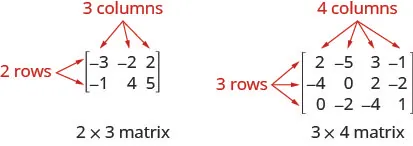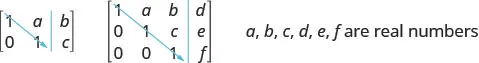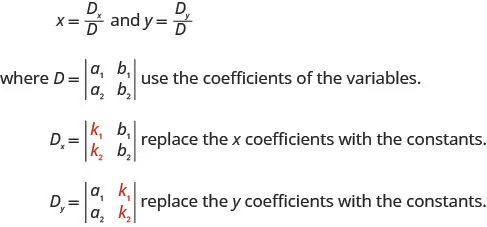Intermediate Algebra 2e

# Key Concepts

### Key Concepts

#### 4.1Solve Systems of Linear Equations with Two Variables

• How to solve a system of linear equations by graphing.
1. Step 1. Graph the first equation.
2. Step 2. Graph the second equation on the same rectangular coordinate system.
3. Step 3. Determine whether the lines intersect, are parallel, or are the same line.
4. Step 4. Identify the solution to the system.
If the lines intersect, identify the point of intersection. This is the solution to the system.
If the lines are parallel, the system has no solution.
If the lines are the same, the system has an infinite number of solutions.
5. Step 5. Check the solution in both equations.
• How to solve a system of equations by substitution.
1. Step 1. Solve one of the equations for either variable.
2. Step 2. Substitute the expression from Step 1 into the other equation.
3. Step 3. Solve the resulting equation.
4. Step 4. Substitute the solution in Step 3 into either of the original equations to find the other variable.
5. Step 5. Write the solution as an ordered pair.
6. Step 6. Check that the ordered pair is a solution to both original equations.
• How to solve a system of equations by elimination.
1. Step 1. Write both equations in standard form. If any coefficients are fractions, clear them.
2. Step 2. Make the coefficients of one variable opposites.
Decide which variable you will eliminate.
Multiply one or both equations so that the coefficients of that variable are opposites.
3. Step 3. Add the equations resulting from Step 2 to eliminate one variable.
4. Step 4. Solve for the remaining variable.
5. Step 5. Substitute the solution from Step 4 into one of the original equations. Then solve for the other variable.
6. Step 6. Write the solution as an ordered pair.
7. Step 7. Check that the ordered pair is a solution to both original equations.
$Choose the Most Convenient Method to Solve a System of Linear EquationsGraphing—————Substitution———————Elimination———————Use when you need apicture of the situation.Use when one equation isalready solved or can beeasily solved for onevariable.Use when the equations arein standard form.Choose the Most Convenient Method to Solve a System of Linear EquationsGraphing—————Substitution———————Elimination———————Use when you need apicture of the situation.Use when one equation isalready solved or can beeasily solved for onevariable.Use when the equations arein standard form.$

#### 4.2Solve Applications with Systems of Equations

• How To Solve Applications with Systems of Equations
1. Step 1. Read the problem. Make sure all the words and ideas are understood.
2. Step 2. Identify what we are looking for.
3. Step 3. Name what we are looking for. Choose variables to represent those quantities.
4. Step 4. Translate into a system of equations.
5. Step 5. Solve the system of equations using good algebra techniques.
6. Step 6. Check the answer in the problem and make sure it makes sense.
7. Step 7. Answer the question with a complete sentence.

#### 4.3Solve Mixture Applications with Systems of Equations

• Cost function: The cost function is the cost to manufacture each unit times x, the number of units manufactured, plus the fixed costs.
$C(x)=(cost per unit)·x+fixed costsC(x)=(cost per unit)·x+fixed costs$
• Revenue: The revenue function is the selling price of each unit times x, the number of units sold.
$R(x)=(sellingpriceperunit)·xR(x)=(sellingpriceperunit)·x$
• Break-even point: The break-even point is when the revenue equals the costs.
$C(x)=R(x)C(x)=R(x)$

#### 4.4Solve Systems of Equations with Three Variables

• Linear Equation in Three Variables: A linear equation with three variables, where a, b, c, and d are real numbers and a, b, and c are not all 0, is of the form
$ax+by+cz=dax+by+cz=d$

Every solution to the equation is an ordered triple, $(x,y,z)(x,y,z)$ that makes the equation true.
• How to solve a system of linear equations with three variables.
1. Step 1. Write the equations in standard form
If any coefficients are fractions, clear them.
2. Step 2. Eliminate the same variable from two equations.
Decide which variable you will eliminate.
Work with a pair of equations to eliminate the chosen variable.
Multiply one or both equations so that the coefficients of that variable are opposites.
Add the equations resulting from Step 2 to eliminate one variable
3. Step 3. Repeat Step 2 using two other equations and eliminate the same variable as in Step 2.
4. Step 4. The two new equations form a system of two equations with two variables. Solve this system.
5. Step 5. Use the values of the two variables found in Step 4 to find the third variable.
6. Step 6. Write the solution as an ordered triple.
7. Step 7. Check that the ordered triple is a solution to all three original equations.

#### 4.5Solve Systems of Equations Using Matrices

• Matrix: A matrix is a rectangular array of numbers arranged in rows and columns. A matrix with m rows and n columns has order $m×n.m×n.$ The matrix on the left below has 2 rows and 3 columns and so it has order $2×3.2×3.$ We say it is a 2 by 3 matrix.Each number in the matrix is called an element or entry in the matrix.
• Row Operations: In a matrix, the following operations can be performed on any row and the resulting matrix will be equivalent to the original matrix.
• Interchange any two rows
• Multiply a row by any real number except 0
• Add a nonzero multiple of one row to another row
• Row-Echelon Form: For a consistent and independent system of equations, its augmented matrix is in row-echelon form when to the left of the vertical line, each entry on the diagonal is a 1 and all entries below the diagonal are zeros.• How to solve a system of equations using matrices.
1. Step 1. Write the augmented matrix for the system of equations.
2. Step 2. Using row operations get the entry in row 1, column 1 to be 1.
3. Step 3. Using row operations, get zeros in column 1 below the 1.
4. Step 4. Using row operations, get the entry in row 2, column 2 to be 1.
5. Step 5. Continue the process until the matrix is in row-echelon form.
6. Step 6. Write the corresponding system of equations.
7. Step 7. Use substitution to find the remaining variables.
8. Step 8. Write the solution as an ordered pair or triple.
9. Step 9. Check that the solution makes the original equations true.

#### 4.6Solve Systems of Equations Using Determinants

• Determinant: The determinant of any square matrix $[abcd],[abcd],$ where a, b, c, and d are real numbers, is
$|abcd|=ad−bc|abcd|=ad−bc$
• Expanding by Minors along the First Row to Evaluate a 3 × 3 Determinant: To evaluate a $3×33×3$ determinant by expanding by minors along the first row, the following pattern:• Sign Pattern: When expanding by minors using a row or column, the sign of the terms in the expansion follow the following pattern.
$|+−+−+−+−+||+−+−+−+−+|$
• Cramer’s Rule: For the system of equations ${a1x+b1y=k1a2x+b2y=k2,{a1x+b1y=k1a2x+b2y=k2,$ the solution $(x,y)(x,y)$ can be determined byNotice that to form the determinant D, we use take the coefficients of the variables.
• How to solve a system of two equations using Cramer’s rule.
1. Step 1. Evaluate the determinant D, using the coefficients of the variables.
2. Step 2. Evaluate the determinant $Dx.Dx.$ Use the constants in place of the x coefficients.
3. Step 3. Evaluate the determinant $Dy.Dy.$ Use the constants in place of the y coefficients.
4. Step 4. Find x and y. $x=DxD,x=DxD,$ $y=DyD.y=DyD.$
5. Step 5. Write the solution as an ordered pair.
6. Step 6. Check that the ordered pair is a solution to both original equations.
7. Step 7. Dependent and Inconsistent Systems of Equations: For any system of equations, where the value of the determinant $D=0,D=0,$
$Value of determinantsType of systemSolutionD=0andDx,DyandDzare all zeroconsistent and dependentinfinitely many solutionsD=0andDx,DyandDzare not all zeroinconsistentno solutionValue of determinantsType of systemSolutionD=0andDx,DyandDzare all zeroconsistent and dependentinfinitely many solutionsD=0andDx,DyandDzare not all zeroinconsistentno solution$
8. Step 8.
Test for Collinear Points: Three points $(x1,y1),(x1,y1),$ $(x2,y2),(x2,y2),$ and $(x3,y3)(x3,y3)$ are collinear if and only if
$|x1y11x2y21x3y31|=0|x1y11x2y21x3y31|=0$

#### 4.7Graphing Systems of Linear Inequalities

• Solutions of a System of Linear Inequalities: Solutions of a system of linear inequalities are the values of the variables that make all the inequalities true. The solution of a system of linear inequalities is shown as a shaded region in the x, y coordinate system that includes all the points whose ordered pairs make the inequalities true.
• How to solve a system of linear inequalities by graphing.
1. Step 1. Graph the first inequality.
Graph the boundary line.
Shade in the side of the boundary line where the inequality is true.
2. Step 2. On the same grid, graph the second inequality.
Graph the boundary line.
Shade in the side of that boundary line where the inequality is true.
3. Step 3. The solution is the region where the shading overlaps.
4. Step 4. Check by choosing a test point.
Order a print copy

As an Amazon Associate we earn from qualifying purchases.